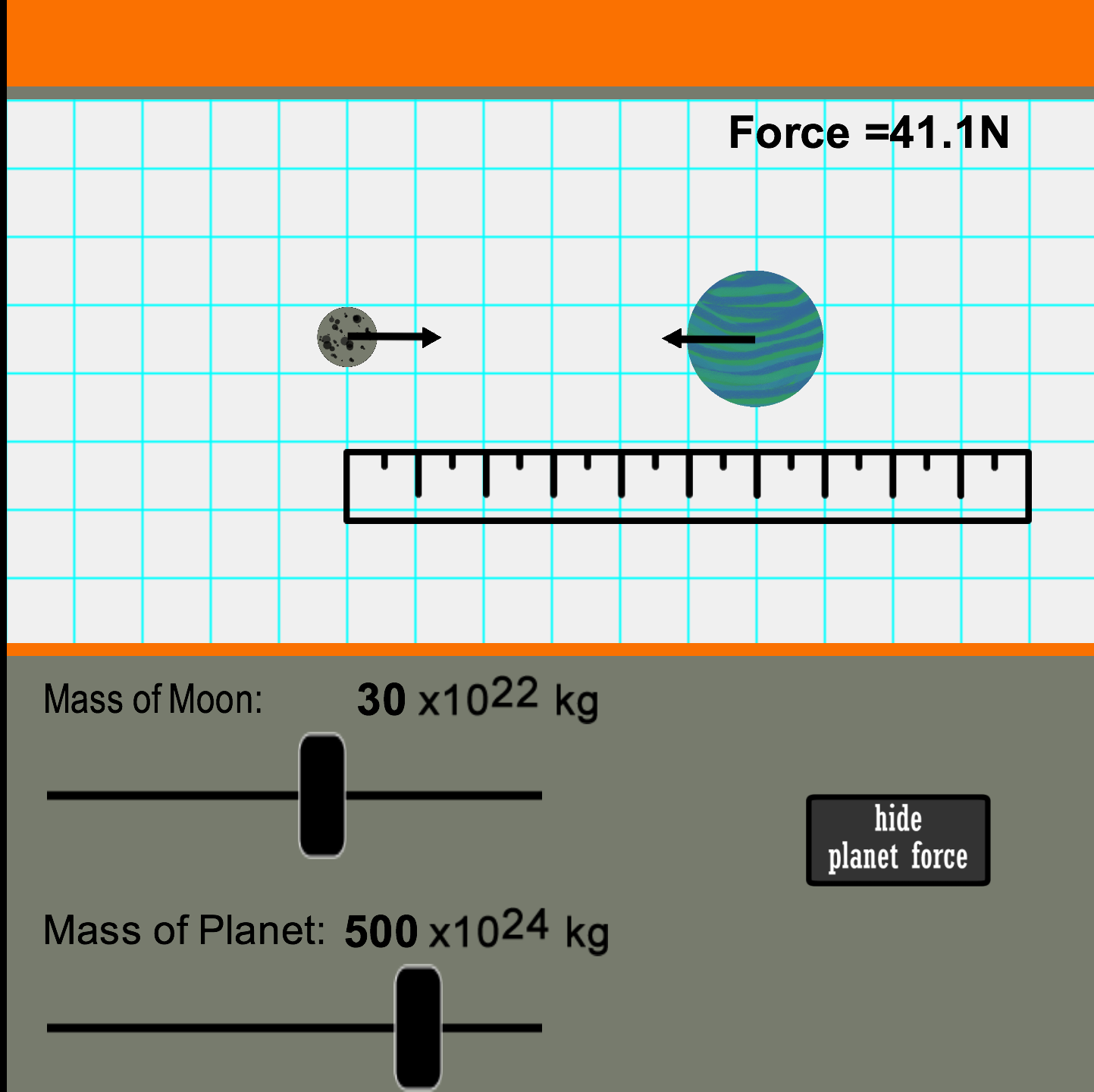###Gravitational Fields

Resource:
Gravitational Fields Interactive

Gravitation – Student Exercise

Description:
The concept of universal gravitation – that all masses attract one another - explains the architecture of the universe. The universal force of gravity follows a very predictable mathematical law. This simulation invites students to explore the mathematics associated with the law in order to learn the variables that affect this very important force.

Performance Expectation:
Force and Motion  (HS-PS2-4):  Use mathematical representations of Newton’s Law of Gravitation to describe the gravitational forces between objects.

This activity aligns with the three dimensions of the Next Generation Science Standards in the manner described below:

Disciplinary Core Ideas
 Types of Interactions (HS-PS2.B.1):  Newton’s Law of Universal Gravitation and Coulomb’s Law provide the mathematical models to describe and predict the effects of gravitational and electrostatic forces between distant objects. The simulation and companion exercise specifically address the first half of this standard in a manner that builds conceptual understanding while also introducing the basic mathematical components of Newton’s Universal Law of Gravitation. The simulation lets learners set the mass of a planet/moon system, then move the objects closer or farther apart and watch the effects on gravitation. The gravitational force is automatically calculated and displayed, allowing students to immediately see how the variables of mass and distance affect the magnitude of force.

Crosscutting Concepts
 Systems and System Models – Grades 9-12:  Models (e.g. physical, mathematical, computer models) can be used to simulate systems and interactions – including energy, matter, and information flows – within and between systems at different scales. Simulations are particularly valuable tools to investigate interactions that occur on the scale of the universe. This model and its companion exercise promote understanding of gravitation by presenting it on a scale students can comprehend. It is similar to the modeling activities undertaken by real astrophysicists to study planetary systems and planet/moon systems from afar. Patterns – Grades 9-12:  Different patterns may be observed at each of the scales at which a system is studied and can provide evidence for causality in explanations of phenomena. This interactive simulation allows students to inspect numerical patterns in universal gravitation data. The result of such an inspection is not only the discovery of the universal gravitation law but also a clear understanding of how changes in one variable affect (or don't affect) another variable.

Practices
 Using Mathematics and Computational Thinking – Grades 9-12:  Use mathematical or computational representations of phenomena to describe explanations. The culminating task of the Student Exercise asks learners to develop an equation with a proportionality constant that describes the relationship between the gravitational force, mass of the two interacting objects (moon and planet), and distance of separation between the objects. Students arrive at such a conclusion after a careful inspection of the simulated data. Constructing Explanations – Grades 9-12:  Apply scientific principles and evidence to provide an explanation of phenomena or solve design problems. The accompanying Student Exercise presents sutdents with the task of determining the quantitative effect of distance on gravitational force, making conclusions about the separation distance between moon-and-planet, and using evidence from the computational model to determine how mass of interacting objects affects gravitational force between them.

Associated Reading from The Physics Classroom

Other Supporting Pages at The Physics Classroom:

View Infographic.
(Coming Soon)

### Search the NGSS Corner

Maybe you're looking for something really specific that pertains to a desired topic and emphasizes one or more of the listed NGSS dimensions. Why not try a search of this section of our website? Simply select from one or more of the pull-down menus and click Search.  This page will reload and a collection  of possibilities will be displayed in this section of the page and sorted by relevancy.